# ECE303L Signals and Systems Lab 5

### Objectives

1. Review properties of operational amplifier.
2. Investigate properties of integrator and differentiator circuits.
3. Investigate properties of a passband Sallen-Key active filter.
4. Investigate the use of frequency sweeps to analyze filter characteristics.

### Background

Download lab5 materials

The pin layout of the LM1458 Dual Operational Amplifier (datasheet) used in this lab is shown in Figure 1 below.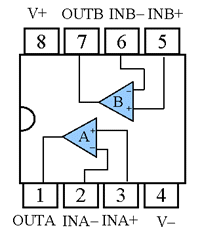Figure 1. LM1458 Pin Diagram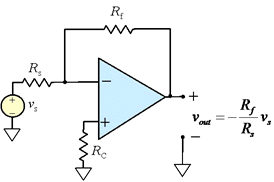Figure 2. Inverting amplifier
Figure 2 shows a simple inverting amplifier circuit that we use to characterize some properties of the operational amplifier itself. Results of some measurements using a different operational amplifier are reported in the link.

### Integrator

An ideal (inverting) Integrator can be build using the schematic in Figure 3 below.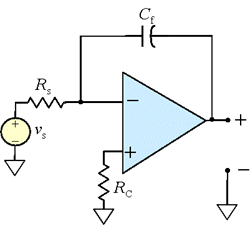Figure 3. Ideal integrator circuit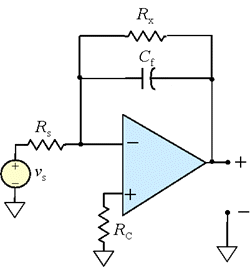Figure 4. Improved integrator circuit.

The ideal integrator suffers from two main limitations. One comes from the fact that the output voltage of the opamp can not exceed the supply voltage. The output of the integrator is inversely proportional to the time constant τ = RsCf. The larger the time constant τ, the longer it takes to saturate the integrator. The second limitation is a consequence of the offset voltage present even for zero input. It may be only a few millivolts, but it it gets integrated over time it eventually drives the OpAmp output to saturation. To prevent this from occurring, a large (1-10 MΩ) resistor Rx is added in parallel with the capacitor, as shown in Figure 4.

### Differentiator

An ideal (inverting) Differentiator can be build using the schematic in Figure 5 below.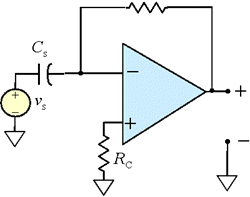Figure 5. Ideal differentiator circuit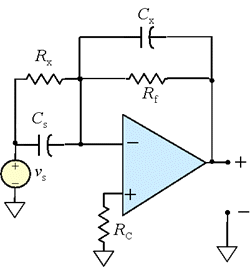Figure 6. Improved differentiator circuit.

Like the integrator before, the ideal differentiator has some limitations. The output is limited to the supply voltages, and since the differentiator is a noise-amplifying device, it suffers from excessive response to high-frequencies. Generally a practical differentiator is combined with a low-pass filter to smooth the high-frequency noise effects. Figure 6 shows some possible modifications to the ideal circuit. A large (over 1 MΩ) resistor Rx may be inserted in parallel with Cs and a very small ( 10 pF or less) capacitor Cx may be inserted in parallel with Rf.

### Sallen-Key Filter

The basic circuit of a band-pass Sallen-Key filter is shown in Figure 7. below.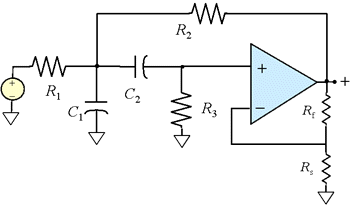Figure 7. Sallen-Key active filter

It can be shown that the transfer function of the filter is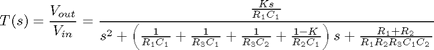Observe that the transfer function shown in equation above is quite complex. The analysis can be greatly simplified, however, if all frequency-determining capacitors are set equal to each other, as well as all frequency-determining resistors. That is, let R1 = R2 = R3/2 = R and C1 = C2 = C. The original transfer function then simplifies to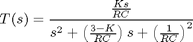A second order band-pass transfer function can written in the following standard form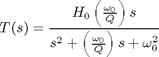Comparing coefficients of the last two equations, we see that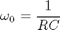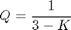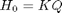An example Sallen-Key bandpass filter, analyzed using MATLAB nodal equations and the symbolic toolkit, is shown the following MATLAB script.

### Frequency Sweep

You learned how to set the function generator to do a frequency sweep in lab 1. It is tempting to analyze an active filter by setting the function generator to sweep through a frequency range of interest and see how the output waveform changes.

An attempt to predict the results of such a measurement resulted in the following MATLAB script. The results were less than satisfactory. The filter examined was a passband filter with center frequency of 1200 Hz and 100 Hz bandwidth. The calculated waveform showed a center frequency of about 1000 Hz and some unexpected structure in the envelope. Either there is a problem with the script or the measurement itself is more complicated than anticipated.

As part of this lab, perform the measurement and report on the results. See if you can find a problem in the MATLAB script.

## Requirements

1. Characterize your operational amplifier by doing the following.
1. Construct the inverting amplifier circuit shown in Figure 1, with a gain of -10. Suggested resistances are Rf = 470 kΩ, Rs = 47 kΩ, and RC = 10 kΩ.
2. Measure the gain and phase shift from 100 Hz to 100 kHz. Use a MATLAB script to make the measurements. Plot the results and tabulate values at decade intervals (100 Hz, 1 kHz, 10 kHz, and 100 kHz).
3. Measure the saturation (clipped) peak-to-peak level at 1 kHz. Set the input so that the ideal output value would be 25 volts peak-to-peak. Attach an image of the oscilloscope output to the report.
4. Measure the slew rate by using a square-wave at a frequency that clearly shows slew-limited behavior. Measure the rise and fall times and the peak-to-peak output voltage. Attach an image of the oscilloscope output to the report.
5. Find the frequency at which the gain drops to 0.707 of its ideal value. Measure the phase shift at that frequency.
6. Ground the input of the OpAmp and measure the output voltage (offset voltage).

2. Build an integrator circuit as shown in Figure 3. Suggested values are 40-100 kΩ for Rs, 100 nF to 0.01 μF for Cf, and 10-20 kΩ for Rc. Try the ideal circuit first and determine the effects of offset voltage by measuring the rate of change of the output voltage with the input grounded. You may need to short the integrating capacitor before starting your measurement. If the output is unstable, insert a large resistor in parallel with the capacitor, as shown in Figure 4. Then characterize the system as follows:
1. Measure the frequency response (magnitude and phase) of the system. Compare the results to theoretical expectations.
2. Show that the output of the integrator is a triangle wave if the input is a square wave. Capture a demonstration oscilloscope trace showing the frequency and peak-to-peak magnitude of input and output.
3. Capture an oscilloscope image showing the output and input of the integrator if the input is a noise signal from the signal generator.

3. Build an differentiator circuit as shown in Figure 5. Suggested values are 40-100 kΩ for Rf, 100 nF to 0.01 μF for Cs, and 10-20 kΩ for Rc. Try the ideal circuit first and determine the effects of noise by evaluating the output for a variety of input signals. If the output noise levels are unacceptable, modify the circuit by the addition of Rx and/or Cx, as shown in Figure 6. Then characterize the system as follows:
1. Measure the frequency response (magnitude and phase) of the system. Compare the results to theoretical expectations.
2. Show that the output of the differentiator is a square wave if the input is a triangle wave. Capture a demonstration oscilloscope trace showing the frequency and peak-to-peak magnitude of input and output.
3. Capture an oscilloscope image showing the output and input of the differentiator if the input is a noise signal from the signal generator.

4. Build a Sallen-Key circuit as shown in Figure 7. Design the filter for a center frequency of 1500 Hz and a Q of 4. Keep resistor values above 1 kΩ and capacitance values above 100 pF. Then characterize the system as follows:
1. Measure the frequency response (magnitude and phase) of the system. Compare the results to theoretical expectations.
2. Calculate the observed values of gain, center frequency, and Q.
3. Capture an oscilloscope image showing the output and input of the filter if the input is a noise signal from the signal generator.

5. Simulate your passband filter in SPICE and compare the results to your measured frequency response above. Adjust the circuit parameters of your SPICE model to match the measured values of your circuit components.

6. Capture an oscilloscope image showing the input and output of the filter above with the function generator set to sweep from 1 kHz to 2 kHz, assuming your passband filter is centered at 1500 Hz. Discuss the resulting image. Does the modulated sweep have its peak value at a time for which the input is the center frequency of the filter? Is the bandwidth of the modulated sweep what you expected? Are there any unexpected structures?

Maintained by John Loomis, last updated 25 October 2010